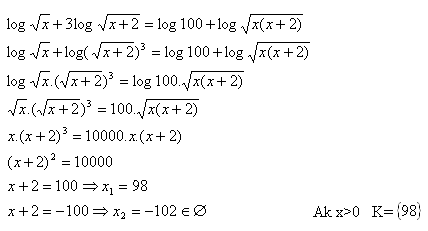sk|cz|

1. Solve:

log(x + 24) + log(x -24) = 2       x > 24
Solution:
log(x + 24) + log(x -24) = 2
log( (x +24)(x – 24)) = log100
(x +24)(x -24)  =  100
x2 – 576  =  100
x2 – 676 = 0
(x – 26)(x + 26) = 0 => x1 = 26 v x2 = -26

K = {26}

2. Solve: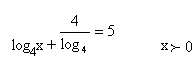Solution: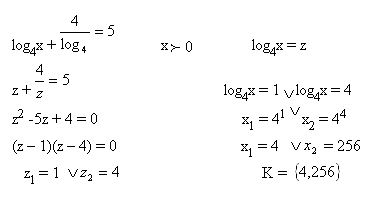3. Solve: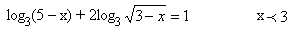Solution: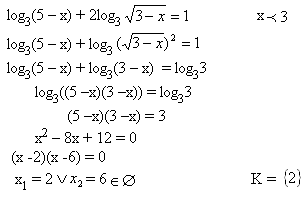4. Solve: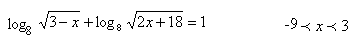Solution: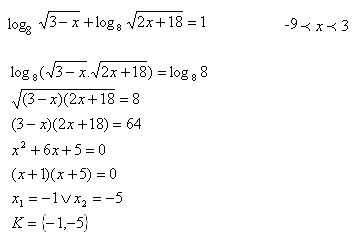5. Solve: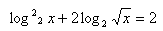Solution:

Substitution: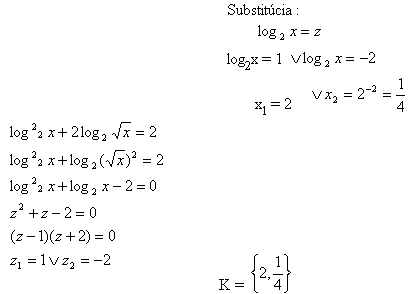6. Solve: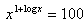Solution: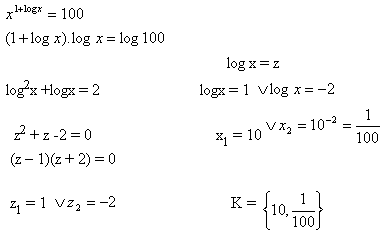7. Solve: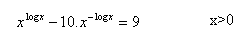Solution: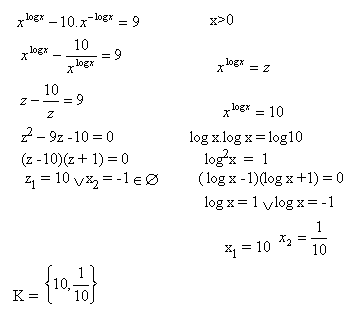8. Solve: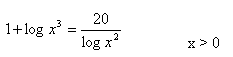Solution: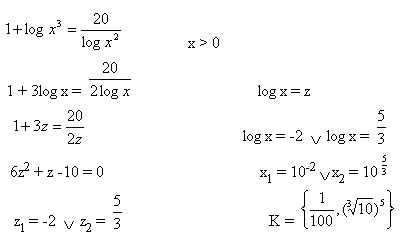9. Solve: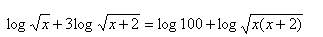Solution: